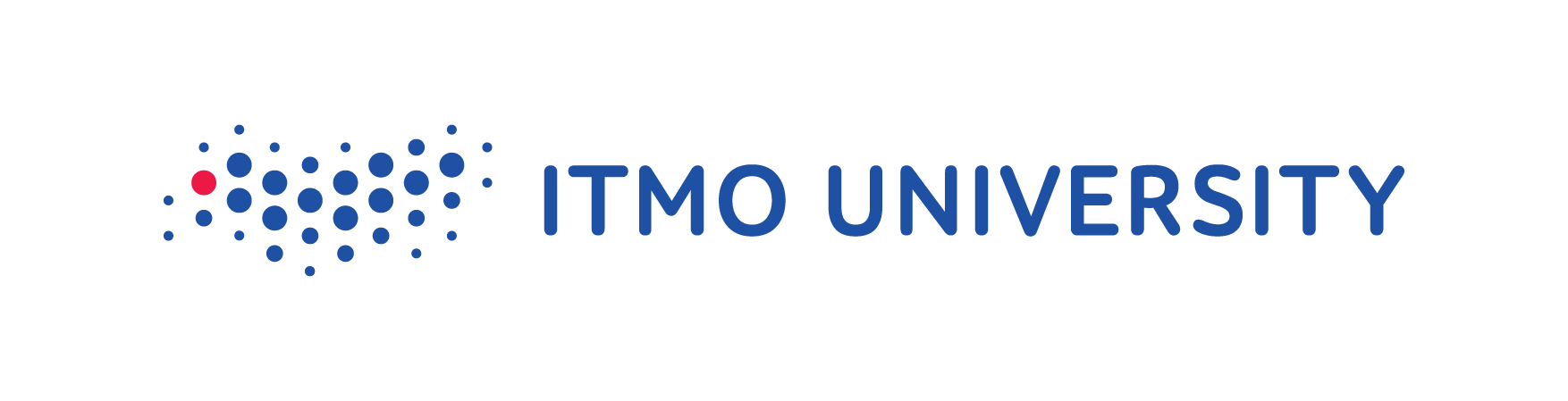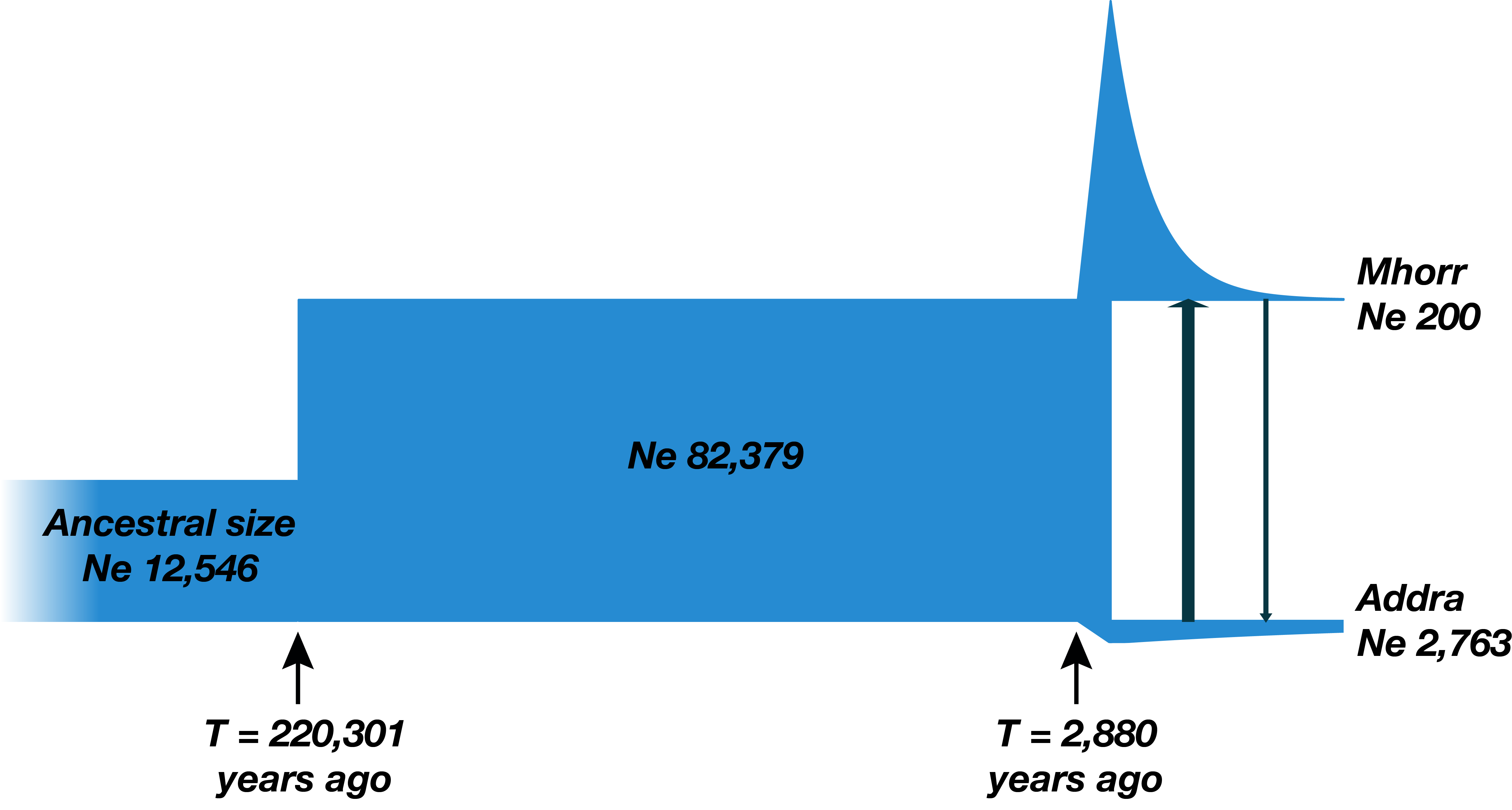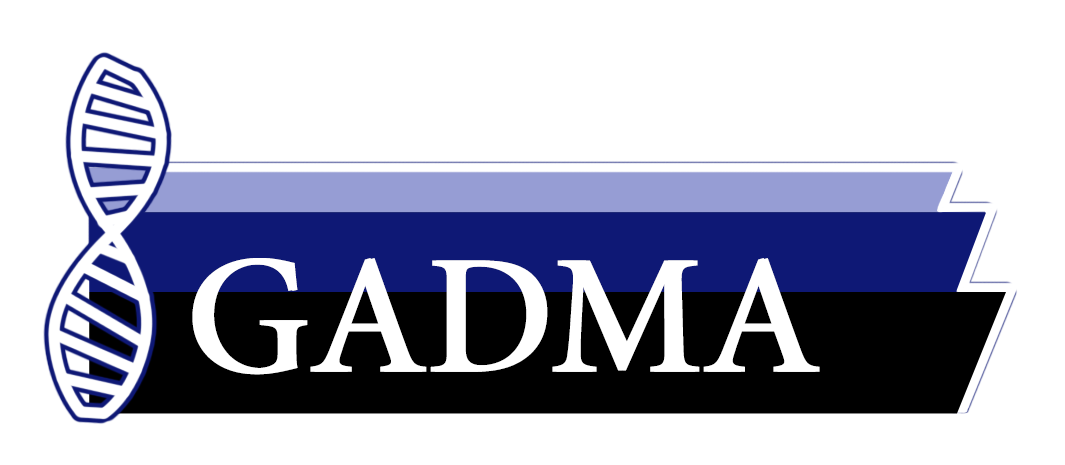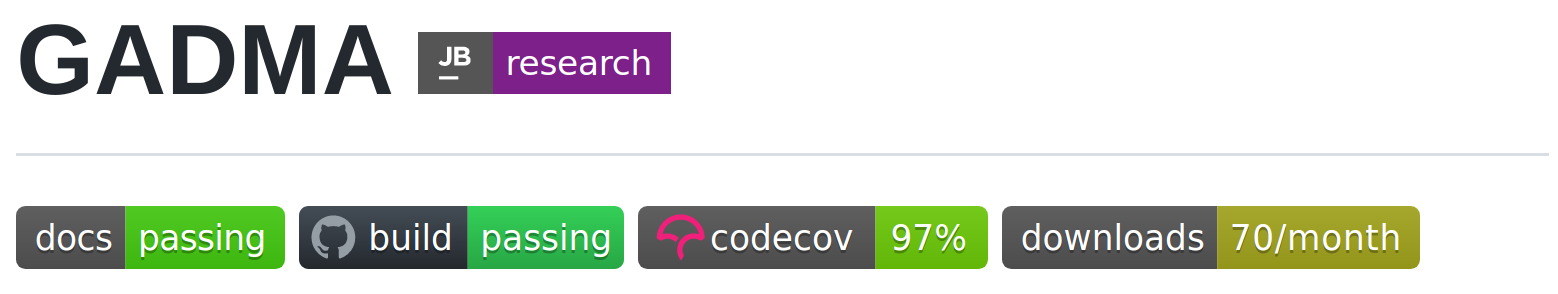Demographic History

Demographic history is the history of evolution and development of populations. It includes such parameters as size of population, time of splits and migration rates.?

Understand population history?

Conservation biology studiesDemographic InferencePipelineExample

$\partial a \partial i$ — Diffusion Approximation for Demographic Inference.$\partial a \partial i$ uses local search optimization algorithms.GADMA — Genetic Algorithm for Demographic Model Analysis

• Several simulation engines ($\partial a \partial i$, moments)
• Has common interface
• Effective optimization based on the genetic algorithm
• Handles up to 3 populationsSimulation Engines Evaluation ComplexityBayesian Optimization

Goal: minimize unknown function $f$ in as few evaluations as possible.

• Black-box optimization
• Expensive evaluations

On each iteration:

• Approximate objective function $f$ with a surrogate model $M$
• Choose next point as $argmax$ of the acquisition function $\alpha_M$ $$\htmlClass{fragment}{ x_{n+1} = \argmax_{x\in\c{X}} \alpha_M(x) }$$

Gaussian ProcessRandom ForestGaussian Process

Definition. A Gaussian process is random function $f : X \to \R$ such that for any $x_1,..,x_n$, the vector $f(x_1),..,f(x_n)$ is multivariate Gaussian.

Every GP is characterized by a mean $\mu(\.)$ and a kernel $k(\.,\.)$. We have $$\htmlClass{fragment}{ f(\v{x}) \~ \f{N}(\v{\mu}_{\v{x}},\m{K}_{\v{x}\v{x}}) }$$ where $\v\mu_{\v{x}} = \mu(\v{x})$ and $\m{K}_{\v{x}\v{x}'} = k(\v{x},\v{x}')$.

Matérn Kernels

$$\htmlData{class=fragment fade-out,fragment-index=9}{ \footnotesize \mathclap{ k_\nu(x,x') = \sigma^2 \frac{2^{1-\nu}}{\Gamma(\nu)} \del{\sqrt{2\nu} \frac{\norm{x-x'}}{\kappa}}^\nu K_\nu \del{\sqrt{2\nu} \frac{\norm{x-x'}}{\kappa}} } } \htmlData{class=fragment d-print-none,fragment-index=9}{ \footnotesize \mathclap{ k_\infty(x,x') = \sigma^2 \exp\del{-\frac{\norm{x-x'}^2}{2\kappa^2}} } }$$ $\sigma^2$: variance $\kappa$: length scale $\nu$: smoothness
$\nu\to\infty$: recovers squared exponential kernel$\nu = 1/2$

Exponential$\nu = 3/2$

Matern32$\nu = 5/2$

Matern52$\nu = \infty$

RBF

Acquisition Functions

• Expected Improvement:

$EI = \mathbb{E}[max\{0, f_{min} - f(x)\}]$

• Probability of Improvement:

$PI = P(f(x) \leq f_{min})$

• Lower Confidence Bound:

$LCB = \mu(x) - \kappa \cdot \sigma(x)$

• Log Expected Improvement:

$logEI = \mathbb{E}[max\{0, e^{f_{min}} - e^{f(x)}\}]$Cross-Validation for Model Selection

The predictive log probability when leaving out training case $(x_i, y_i)$ is: $$\htmlClass{fragment}{ \footnotesize \mathclap{ \log p(y_i | X, y_{-i}, \theta) = -\frac{1}{2} \log \sigma_i^2 - \frac{(y_i - \mu_i)^2}{2\sigma^2} - \frac{1}{2}\log 2\pi, } }$$ where $\theta$ - parameters of Gaussian Process, $y_{-i} = Y \setminus \{y_i\}$, $\mu_i = \mu(x_i)$ and $\sigma_i = \sigma(x_i)$

Leave-One-Out log predictive probability: $$\htmlClass{fragment}{ L_{LOO} = \sum_{i=1}^n \log p(y_i | X, y_{-i}, \theta) }$$

DatasetsDatasetsBayesian Optimization PerformanceBayesian Optimization PerformanceAutomatic Kernel Selection and EnsemblingAutomatic Kernel Selection and EnsemblingConclusions

• Performance depends on kernel and acquisition function.
• Leave-one-out cross-validation:
• poor for kernel selection based on large random dataset,
• good for automatic kernel selection at the beginning.
• Bayesian optimization with automatic kernel choice showed faster convergence than the genetic algorithm for 4 and 5 populations.

Future Work

• Combine Bayesian optimization and genetic algorithm.
• Stop criteria for Bayesian optimization.
• More experiments on real data.

AcknowledgementsViacheslavBorovitskiyPavelDobrynin